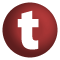# Show

#### trait Show extends AnyRef

Classes inheriting trait `Show` can test their member methods using the notattion `meth(arg1, ..., argn)`, where `meth` is the name of the method and `arg1,...,argn` are the arguments. The only difference to a normal method call is the leading quote character ('). A quoted method call like the one above will produces a legible diagnostic to be printed on `Console`. It is of the form

`   meth(arg1, ..., argn)  gives  <result>`

where `<result>` is the result of evaluating the call.

1. AnyRef
2. Any

### Type Members

1. #### class SymApply extends AnyRef

The result class of wrapper `symApply`

### Value Members

1. #### def equals(arg0: Any): Boolean

This method is used to compare the receiver object (`this`) with the argument object (`arg0`) for equivalence

This method is used to compare the receiver object (`this`) with the argument object (`arg0`) for equivalence.

The default implementations of this method is an equivalence relation:

• It is reflexive: for any instance `x` of type `Any`, `x.equals(x)` should return `true`.
• It is symmetric: for any instances `x` and `y` of type `Any`, `x.equals(y)` should return `true` if and only if `y.equals(x)` returns `true`.
• It is transitive: for any instances `x`, `y`, and `z` of type `AnyRef` if `x.equals(y)` returns `true` and `y.equals(z)` returns `true`, then `x.equals(z)` should return `true`.

If you override this method, you should verify that your implementation remains an equivalence relation. Additionally, when overriding this method it is often necessary to override `hashCode` to ensure that objects that are "equal" (`o1.equals(o2)` returns `true`) hash to the same `Int` (`o1.hashCode.equals(o2.hashCode)`).

arg0

the object to compare against this object for equality.

returns

`true` if the receiver object is equivalent to the argument; `false` otherwise.

definition classes: AnyRef ⇐ Any
2. #### def hashCode(): Int

Returns a hash code value for the object

Returns a hash code value for the object.

The default hashing algorithm is platform dependent.

Note that it is allowed for two objects to have identical hash codes (`o1.hashCode.equals(o2.hashCode)`) yet not be equal (`o1.equals(o2)` returns `false`). A degenerate implementation could always return `0`. However, it is required that if two objects are equal (`o1.equals(o2)` returns `true`) that they have identical hash codes (`o1.hashCode.equals(o2.hashCode)`). Therefore, when overriding this method, be sure to verify that the behavior is consistent with the `equals` method.

definition classes: AnyRef ⇐ Any
3. #### def symApply(sym: Symbol): SymApply

An implicit definition that adds an apply method to Symbol which forwards to `test`

An implicit definition that adds an apply method to Symbol which forwards to `test`.

attributes: implicit
4. #### def test[A](f: Symbol, args: A*): String

Apply method with name of given symbol `f` to given arguments and return a result diagnostics

Apply method with name of given symbol `f` to given arguments and return a result diagnostics.

5. #### def toString(): String

Returns a string representation of the object

Returns a string representation of the object.

The default representation is platform dependent.

definition classes: AnyRef ⇐ Any